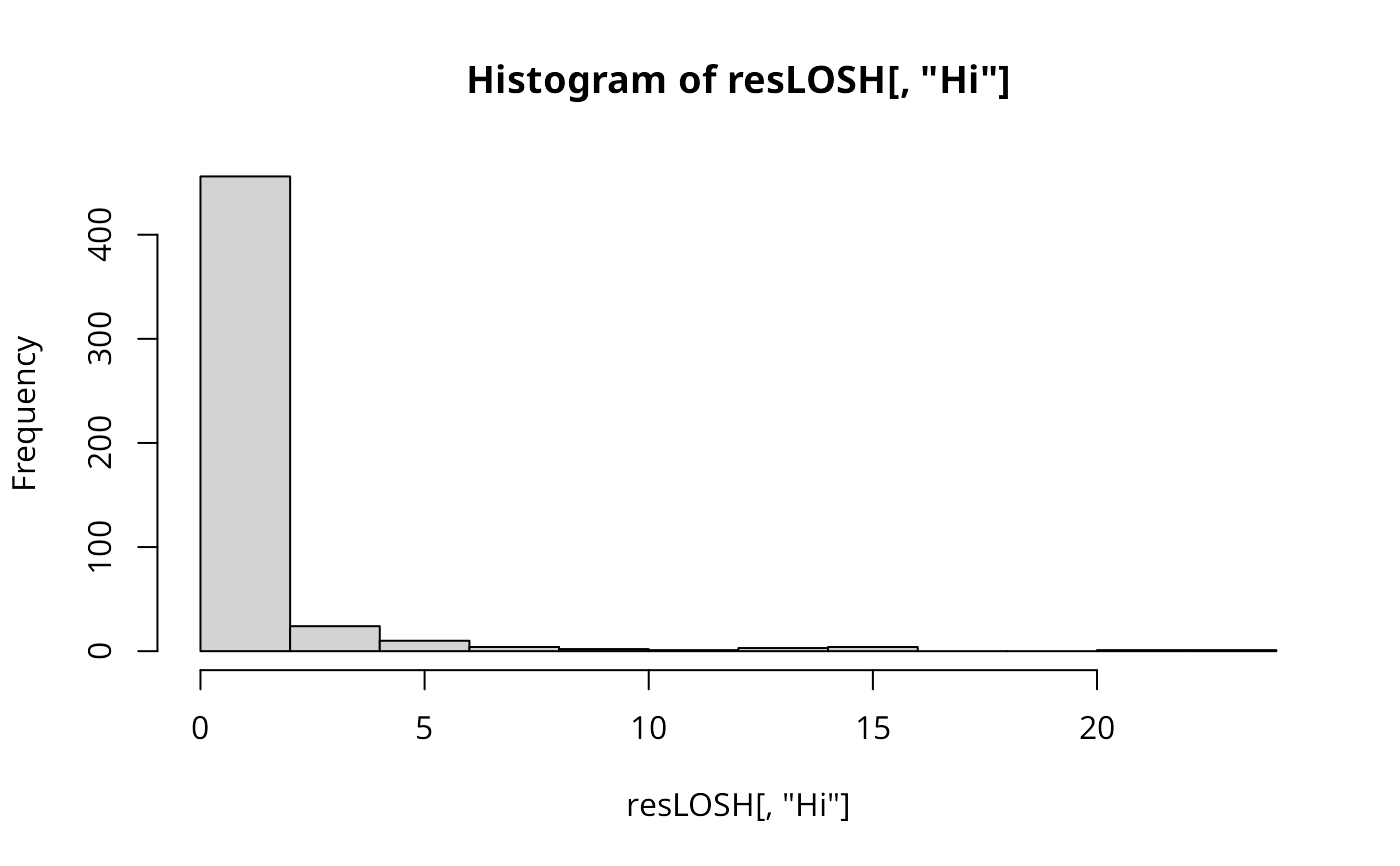Local spatial heteroscedasticity is calculated for each location based on the spatial weights object used. The statistic is: $$H_i = \frac{\sum_j^n w_{ij} \cdot |e_j|^a}{h_1 \cdot \sum_j^n w_{ij}}$$ with $$e_j = x_j - \bar{x}_j$$ and $$\bar{x}_j = \frac{\sum_k^n w_{jk} \cdot x_k}{\sum_k^n w_{jk}}$$ Its expectation and variance are given in Ord & Getis (2012). The exponent a allows for investigating different types of mean dispersal.

LOSH(x, listw, a=2, var_hi=TRUE, zero.policy=NULL, na.action=na.fail, spChk=NULL)

## Arguments

x

a numeric vector of the same length as the neighbours list in listw

listw

a listw object created for example by nb2listw

a

the exponent applied to the local residuals; the default value of 2 leads to a measure of heterogeneity in the spatial variance

var_hi

default TRUE, the moments and the test statistics are calculated for each location; if FALSE, only the plain LOSH measures, $$\bar{x}_i$$ and $$e_i$$ are calculated

zero.policy

default NULL, use global option value; if TRUE assign zero to the lagged value of zones without neighbours, if FALSE assign NA

na.action

a function (default na.fail), can also be na.omit or na.exclude - in these cases the weights list will be subsetted to remove NAs in the data. It may be necessary to set zero.policy to TRUE because this subsetting may create no-neighbour observations. Note that only weights lists created without using the glist argument to nb2listw may be subsetted. If na.pass is used, zero is substituted for NA values in calculating the spatial lag. (Note that na.exclude will only work properly starting from R 1.9.0, na.omit and na.exclude assign the wrong classes in 1.8.*)

spChk

should the data vector names be checked against the spatial objects for identity integrity, TRUE, or FALSE, default NULL to use get.spChkOption()

## Details

In addition to the LOSH measure, the values returned include local spatially weighted mean values $$\bar{x}_i$$ and local residuals $$e_i$$ estimated about these means. These values facilitate the interpretation of LOSH values. Further, if specified through var_hi, the statistical moments and the test statistics as proposed by Ord & Getis (2012) are also calculated and returned.

## Value

Hi

LOSH statistic

E.Hi

(optional) expectation of LOSH

Var.Hi

(optional) variance of LOSH

Z.Hi

(optional) the approximately Chi-square distributed test statistics

x_bar_i

local spatially weighted mean values

ei

residuals about local spatially weighted mean values

## Author

René Westerholt rene.westerholt@tu-dortmund.de

LOSH.cs, LOSH.mc
    data(boston, package="spData")